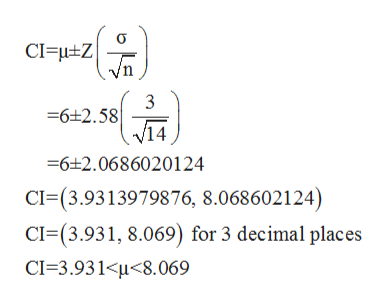# Suppose that 14 children, who were learning to ride two-wheel bikes, were surveyed to determine how long they had to use training wheels. It was revealed that they used them an average of six months with a sample standard deviation of three months. Assume that the underlying population distribution is normal.Construct a 99% confidence interval for the population mean length of time using training wheels.What is the lower bound? (Round to 3 decimal places)What is the upper bound? (Round to 3 decimal places)

Question
160 views

Suppose that 14 children, who were learning to ride two-wheel bikes, were surveyed to determine how long they had to use training wheels. It was revealed that they used them an average of six months with a sample standard deviation of three months. Assume that the underlying population distribution is normal.

Construct a 99% confidence interval for the population mean length of time using training wheels.

What is the lower bound? (Round to 3 decimal places)

What is the upper bound? (Round to 3 decimal places)

check_circle

Step 1

Given information is below,

Sample size (n) = 14,

Mean (μ) = 6 months,

Population standard deviation (σ) = 3 months.

Step 2

Formula for confidence interval for population mean is,

Step 3

Now, substitute given values...help_outlineImage TranscriptioncloseCI HZ 6 2.58 /14 6 2.0686020124 CI (3.9313979876, 8.068602124) CI-(3.931, 8.069) for 3 decimal places CI-3.931

### Want to see the full answer?

See Solution

#### Want to see this answer and more?

Solutions are written by subject experts who are available 24/7. Questions are typically answered within 1 hour.*

See Solution
*Response times may vary by subject and question.
Tagged in

### Statistics